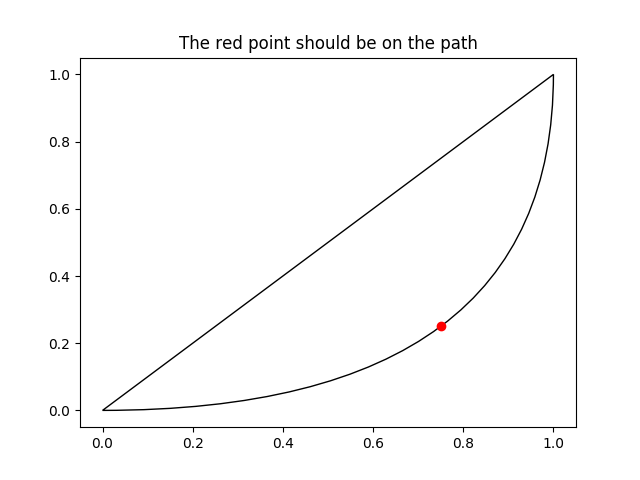# Bezier Curve¶

This example showcases the `PathPatch` object to create a Bezier polycurve path patch.

```import matplotlib.path as mpath
import matplotlib.patches as mpatches
import matplotlib.pyplot as plt

Path = mpath.Path

fig, ax = plt.subplots()
pp1 = mpatches.PathPatch(
Path([(0, 0), (1, 0), (1, 1), (0, 0)],
[Path.MOVETO, Path.CURVE3, Path.CURVE3, Path.CLOSEPOLY]),
fc="none", transform=ax.transData)